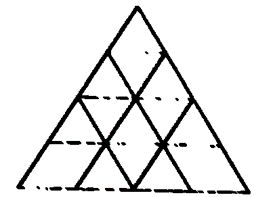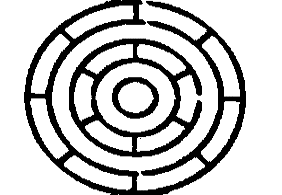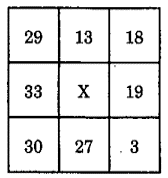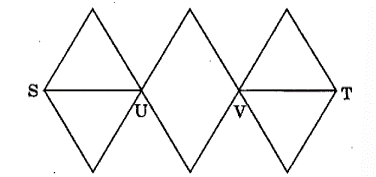# Logical Ability UPSC Questions

## Logical Ability MCQ Questions

1.

Consider the following figure and answer the item that follows:What is the total number of triangles in the above grid?

A.

27

B.

26

C.

23

D.

22

C. 23

2.

Consider the following figure and answer the item that follows:What is the minimum number of different colours required to paint the figure given above such that no two adjacent regions have the same colour?

UPSC - 2011

A.

6

B.

3

C.

4

D.

5

B. 3

3.

Consider that:
1. A is taller than B.
2. C is taller than A.
3. D is taller than C.
4. E is the tallest of all.
If they are made to sit in the above order of their height, who will occupy the mid position?

UPSC - 2014

A.

A

B.

B

C.

C

D.

D

C. C

4.

Consider the table given below in which the numbers bear certain relationship among themselves along the rows:Which one of the following numbers is the missing number indicated above by X ?

UPSC - 2014

A.

19

B.

15

C.

14

D.

8

B. 15

5.

With reference to the figure given below, the number of different routes from S to T without retracing from U and/or V, isUPSC - 2014

A.

3

B.

6

C.

9

D.

18

D. 18

6.

The letters L, M, N, O, P, Q, R, S and T in their order are substituted by nine integers 1 to 9 but not in that order. 4 is assigned to P. The difference between P and T is 5. The difference between N and T is 3. What is the integer assigned to N?

UPSC - 2014

A.

7

B.

5

C.

4

D.

6